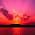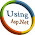# Introduction: Hello guys, in this article i will explain that how we can createVisualization: Combo Chartusing asp.net. This is very helpful artucle for the asp.net deveploer to creating a sexy graph in asp.net.

Implementation: Here i am taking the reference from the below link . http://code.google.com/apis/chart/interactive/docs/gallery/combochart.html
Google use javascript code for the implementation of this graph. I am using same javascript code but am mixing my asp.net experience with this code. And create the same graph in Asp.Net.

Code for .aspx page:
<meta http-equiv="content-type" content="text/html; charset=utf-8"/>
<title>
</title>
<%--the below two javascripts  are needed to run this program you can

<script type="text/javascript">
</script>
<body>
<form id="form1" runat="server">
<div>
<%--Here am taking Literal control from the toolbox --%>
<asp:Literal ID="lt" runat="server"></asp:Literal>
</div>
<div id="chart_div" style="width: 700px; height: 400px;"></div>

</form>
</body>
Code for aspx.cs page:

using System;
using System.Collections;
using System.Configuration;
using System.Data;
using System.Linq;
using System.Web;
using System.Web.Security;
using System.Web.UI;
using System.Web.UI.HtmlControls;
using System.Web.UI.WebControls;
using System.Web.UI.WebControls.WebParts;
using System.Xml.Linq;
using System.Data.SqlClient;
using System.Text;

public partial class complete_graph_without_classes : System.Web.UI.Page
{
// complete code of Visualization: Combo Chart
SqlConnection con = new SqlConnection();
// here am declairing the stringbuilder class
StringBuilder str = new StringBuilder();
protected void Page_Load(object sender, EventArgs e)
{
// here i am declare connection
con.ConnectionString = ConfigurationManager.ConnectionStrings["cn"].ConnectionString;
con.Open();
if (Page.IsPostBack == false)
{
// here i am calling function  chart_bind(); in the page load event of the page
chart_bind();
}

}
private void chart_bind()
{
// here i am using SqlDataAdapter for the sql server select query
// here am taking datatable
DataTable dt = new DataTable();
try
{
// here datatale dt is fill wit the adp
// this string m catching in the stringbuilder class
// in the str m writing same javascript code that is given by the google.
// but m changing  only below line
// ['Student Name', 'Science', 'Math', 'Social Science', 'Hindi', 'english', 'punjabi'],"); according to
// my data that will come from the sql server
str.Append(@"<script type=text/javascript>
function drawVisualization() {
// Some raw data (not necessarily accurate)
['Student Name', 'Science', 'Math', 'Social Science', 'Hindi', 'english', 'punjabi'],");
// here i am declairing the variable i in int32 for the looping statement
Int32 i;
// loop start from 0 and its end depend on the value inside dt.Rows.Count - 1
for (i = 0; i <= dt.Rows.Count - 1; i++)
{
// here i am fill the string builder with the value from the database
str.Append("['" + (dt.Rows[i]["student_name"].ToString()) + "'," + dt.Rows[i]["science"].ToString() + "," + dt.Rows[i]["math"].ToString() + "," + dt.Rows[i]["social_science"].ToString() + "," + dt.Rows[i]["hindi"].ToString() + "," + dt.Rows[i]["english"].ToString() + "," + dt.Rows[i]["punjabi"].ToString() + "],");
}
// other all string is fill according to the javascript code
str.Append("  ]);");
str.Append("  var comboChart = new google.visualization.ComboChart(document.getElementById('chart_div'));");
str.Append(" comboChart.draw(data, {");
str.Append(" title : 'Students Report Card',");
str.Append("vAxis: {title:" + "*Marks in diffrent subjects*" + "},");
str.Append(" hAxis: {title:" + "*Student Name*" + " },");
str.Append("seriesType:" + "*bars*" + " ,");
// in the below line i need " in place of *
// stringbuilder can't return us " so at the last line i am
// replacing * with the " using Replace('*', '"'); function
// and other code is same like the google code
str.Append("series: {" + dt.Rows.Count + ": {type: " + "*line*" + "}}");
str.Append("}); }");
str.Append("</script>");
// here am using literal conrol to display the complete graph
lt.Text = str.ToString().TrimEnd(',').Replace('*', '"');
con.Close();
}
catch
{ }

}
}
See output in this image:

Sql Script for database:

/****** Object:  Table [dbo].[tb_all_subject_record]    Script Date: 07/02/2011 16:31:36 ******/
IF  EXISTS (SELECT * FROM sys.objects WHERE object_id = OBJECT_ID(N'[dbo].[tb_all_subject_record]') AND type in (N'U'))
DROP TABLE [dbo].[tb_all_subject_record]
GO
/****** Object:  Table [dbo].[tb_all_subject_record]    Script Date: 07/02/2011 16:31:36 ******/
SET ANSI_NULLS ON
GO
SET QUOTED_IDENTIFIER ON
GO
IF NOT EXISTS (SELECT * FROM sys.objects WHERE object_id = OBJECT_ID(N'[dbo].[tb_all_subject_record]') AND type in (N'U'))
BEGIN
CREATE TABLE [dbo].[tb_all_subject_record](
[id] [int] IDENTITY(1,1) NOT NULL,
[student_name] [varchar](50) COLLATE SQL_Latin1_General_CP1_CI_AS NULL,
[science] [varchar](50) COLLATE SQL_Latin1_General_CP1_CI_AS NULL,
[math] [varchar](50) COLLATE SQL_Latin1_General_CP1_CI_AS NULL,
[social_science] [varchar](50) COLLATE SQL_Latin1_General_CP1_CI_AS NULL,
[hindi] [varchar](50) COLLATE SQL_Latin1_General_CP1_CI_AS NULL,
[english] [varchar](50) COLLATE SQL_Latin1_General_CP1_CI_AS NULL,
[punjabi] [varchar](50) COLLATE SQL_Latin1_General_CP1_CI_AS NULL,
CONSTRAINT [PK_tb_all_subject_record] PRIMARY KEY CLUSTERED
(
[id] ASC
)WITH (PAD_INDEX  = OFF, STATISTICS_NORECOMPUTE  = OFF, IGNORE_DUP_KEY = OFF, ALLOW_ROW_LOCKS  = ON, ALLOW_PAGE_LOCKS  = ON)
)
END
GO
SET IDENTITY_INSERT [dbo].[tb_all_subject_record] ON
INSERT [dbo].[tb_all_subject_record] ([id], [student_name], [science], [math], [social_science], [hindi], [english], [punjabi]) VALUES (1, N'vikas', N'15', N'25', N'56', N'45', N'56', N'52')
INSERT [dbo].[tb_all_subject_record] ([id], [student_name], [science], [math], [social_science], [hindi], [english], [punjabi]) VALUES (2, N'raman', N'23', N'25', N'26', N'53', N'38', N'45')
INSERT [dbo].[tb_all_subject_record] ([id], [student_name], [science], [math], [social_science], [hindi], [english], [punjabi]) VALUES (3, N'bharat', N'56', N'56', N'54', N'58', N'48', N'35')
INSERT [dbo].[tb_all_subject_record] ([id], [student_name], [science], [math], [social_science], [hindi], [english], [punjabi]) VALUES (4, N'vikram', N'54', N'58', N'59', N'63', N'36', N'53')
INSERT [dbo].[tb_all_subject_record] ([id], [student_name], [science], [math], [social_science], [hindi], [english], [punjabi]) VALUES (5, N'puneet', N'23', N'25', N'25', N'56', N'44', N'35')
INSERT [dbo].[tb_all_subject_record] ([id], [student_name], [science], [math], [social_science], [hindi], [english], [punjabi]) VALUES (6, N'akash', N'23', N'32', N'34', N'89', N'57', N'87')
INSERT [dbo].[tb_all_subject_record] ([id], [student_name], [science], [math], [social_science], [hindi], [english], [punjabi]) VALUES (7, N'manav', N'76', N'32', N'66', N'45', N'57', N'62')
INSERT [dbo].[tb_all_subject_record] ([id], [student_name], [science], [math], [social_science], [hindi], [english], [punjabi]) VALUES (8, N'amar', N'25', N'87', N'44', N'44', N'57', N'38')
INSERT [dbo].[tb_all_subject_record] ([id], [student_name], [science], [math], [social_science], [hindi], [english], [punjabi]) VALUES (9, N'preeti', N'68', N'87', N'90', N'44', N'20', N'30')
INSERT [dbo].[tb_all_subject_record] ([id], [student_name], [science], [math], [social_science], [hindi], [english], [punjabi]) VALUES (10, N'namita', N'34', N'56', N'66', N'37', N'32', N'84')
INSERT [dbo].[tb_all_subject_record] ([id], [student_name], [science], [math], [social_science], [hindi], [english], [punjabi]) VALUES (11, N'heena', N'66', N'52', N'20', N'38', N'12', N'47')
INSERT [dbo].[tb_all_subject_record] ([id], [student_name], [science], [math], [social_science], [hindi], [english], [punjabi]) VALUES (12, N'pooja', N'34', N'90', N'23', N'20', N'28', N'47')
INSERT [dbo].[tb_all_subject_record] ([id], [student_name], [science], [math], [social_science], [hindi], [english], [punjabi]) VALUES (13, N'rajjo', N'23', N'45', N'89', N'21', N'39', N'85')
INSERT [dbo].[tb_all_subject_record] ([id], [student_name], [science], [math], [social_science], [hindi], [english], [punjabi]) VALUES (14, N'manpreet', N'23', N'20', N'54', N'53', N'23', N'89')
INSERT [dbo].[tb_all_subject_record] ([id], [student_name], [science], [math], [social_science], [hindi], [english], [punjabi]) VALUES (15, N'vaneet', N'45', N'78', N'09', N'02', N'85', N'89')
INSERT [dbo].[tb_all_subject_record] ([id], [student_name], [science], [math], [social_science], [hindi], [english], [punjabi]) VALUES (16, N'dushyant', N'45', N'32', N'56', N'80', N'85', N'60')
INSERT [dbo].[tb_all_subject_record] ([id], [student_name], [science], [math], [social_science], [hindi], [english], [punjabi]) VALUES (17, N'divya', N'45', N'32', N'89', N'28', N'85', N'65')
INSERT [dbo].[tb_all_subject_record] ([id], [student_name], [science], [math], [social_science], [hindi], [english], [punjabi]) VALUES (18, N'meenu', N'00', N'32', N'89', N'28', N'85', N'00')
SET IDENTITY_INSERT [dbo].[tb_all_subject_record] OFF
Conclusion
Through this article, you have learned how to create Visualization: Combo Chart using the google’s javascript code as well as asp.net code.

1.Hello,

This Chart Is Not Working On IE Browser Version 8.0 +.

Its Give Error Array Null Or Dt Not Fill.
So, Check It and Give Reply..

Thanks

1.On the my end this code is running properly.Debug it properly when you will run this code and also check your database that there is values available inside the table or not... and if you want to increase or decrease the subjects from the table then set the code properly after studying..

Thankx..

regards
Bharat Bhushan

2.3.